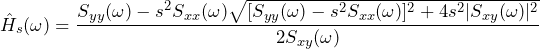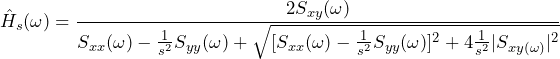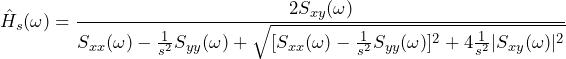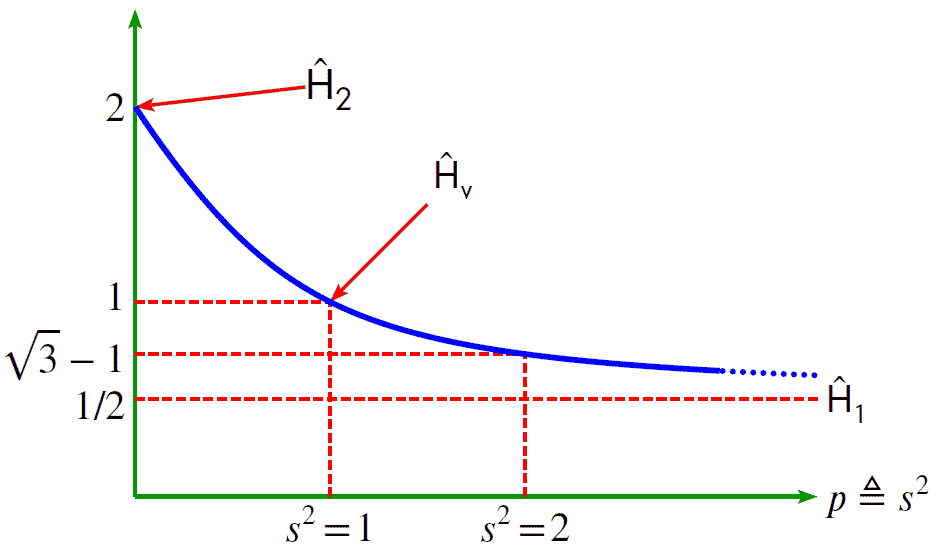# Scaled Transfer Function Estimator

June 2, 2021

The scaled transfer function estimator Ĥs(f) with scaling factor s (where 0 ≤ s ≤ ∞) is an estimate of the true transfer function H(f) of a system.

The scaling factor is the ratio of output noise power to input noise power such that s2 = Ŝyyxx. This is with the assumption that Ŝxx(f) and Ŝyy(f) are constant with respect to frequency f.

Ĥs is a generalization of the transfer function estimators Ĥ1, Ĥ2, and Ĥv. Ĥs is defined as:

(1)### Alternate Forms

Using the technique of rationalizing the denominator—or, in this case, rationalizing the numerator—we can arrive at alternate and useful forms for Ĥs. Particularly, if we multiply numerator and denominator by the rationalizing factor, we can arrive at the following equation:

(2)Moreover, if we divide the numerator and denominator by s2, we get:

(3)### Special Cases of Ĥs

Ĥ1, Ĥ2, and Ĥv are special cases of Ĥs. Using the definition of Ĥs, we see Ĥs=0=Ĥ2 and Ĥs=1=Ĥv. Using the second alternate form, we see Ĥs➜∞=Ĥ1.